Home | | Chemistry 12th Std | Chemical Kinetics: Multiple choice questions with answers

# Chemical Kinetics: Multiple choice questions with answers

Chemistry : Chemical Kinetics: Multiple choice questions with answers, Solution and Explanation

Chemical Kinetics

EVALUATION

1. For a first order reaction A → B the rate constant is x min1 . If the initial concentration of A is 0.01M , the concentration of A after one hour is given by the expression.

a) 0.01 e−x

b) 1 x10-2 (1-e-60x)

(c) (1x10-2) e-60x

d) none of these

SolutionIn this case

k = x min−1 and [A0] = 0.01M = 1 × 10−2M

t = 1 hour = 60 min

[A]= 1 × 10−2 ( e−60x)

2. A zero order reaction X →Product , with an initial concentration 0.02M has a half life of 10 min. if one starts with concentration 0.04M, then the half life is

a) 10 s

b) 5 min

c) 20 min

d) cannot be predicted using the given information

Solution3. Among the following graphs showing variation of rate constant with temperature (T) for a reaction, the one that exhibits Arrhenius behavior over the entire temperature range isd) both (b) and (c)

Ans: (b)

Solution

k = A e –(Ea/RT)

ln k = ln A – (Ea/R)  (1/T)

this equation is in the form of a straight line equatoion

y = c + m x

a plot of lnk vs 1/T is a straight line with negative slope

4. For a first order reaction A → product with initial concentration x mol L1 , has a half life period of 2.5 hours . For the same reaction with initial concentration (x/2) mol L1 the half life is

a) (2.5 × 2) hours

b) (2.5 / 2) hours

c) 2.5 hours

d) Without knowing the rate constant, t1/2 cannot be determined from the given data

Solution

For a first order reaction

t1/2 = 0.693/k

t1/2 does not depend on the initial concentration and it remains constant (whatever may be the initial concentration)

t1/2 = 2.5 hrs

5. For the reaction, 2NH3 → N2 + 3H2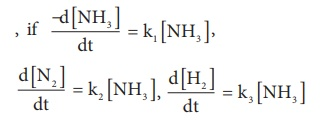then the relation between k1, k2 and k3 is

a) k1 = k2 = k3

b) k1 = 3k2 = 2k3

c) 1.5 k1 = 3 k2 = k3

d) 2 k1 = k2 = 3 k3

Solution(3/2)k1 =  3k2 = k3

1.5 k1 = 3k2 = k3

6. The decomposition of phosphine (PH3) on tungsten at low pressure is a first order reaction. It is because the

a) rate is proportional to the surface coverage

b) rate is inversely proportional to the surface coverage

c) rate is independent of the surface coverage

d) rate of decomposition is slow

Solution

At low pressure the reaction follows first order, therefore

Rate α [reactant]1

Rate α ( surface area )

At high pressure due to the complete coverage of surface area, the reaction follows zero order.

Rate α[reactant]0

Therefore the rate is independent of surface area.

7. For a reaction Rate = k[acetone]3/2 acetone then unit of rate constant and rate of reaction respectively is

a) (mol L-1s-1), (mol-1/2 L1/2 s-1)

b) (mol-1/2 L1/2s-1), (mol L-1 s-1)

c) (mol1/2 L1/2s-1), (mol L-1 s-1)

d) (mol Ls-1), (mol1/2 L1/2 s-1)

Solution8. The addition of a catalyst during a chemical reaction alters which of the following quantities?

a) Enthalpy

b)Activation energy

c) Entropy

d) Internal energy

Solution

A catalyst provides a new path to the reaction with low activation energy. i.e., it lowers the activation energy.

9. Consider the following statements :

(i) increase in concentration of the reactant increases the rate of a zero order reaction.

(ii) rate constant k is equal to collision frequency A if Ea = 0

(iii) rate constant k is equal to collision frequency A if Ea = °

(iv) a plot of ln(k) vs T is a straight line

(v) a plot of ln (k) vs 1/T  is a straight line with a positive slope.

Correct statements are

a) (ii) only

b) (ii) and (iv)

c) (ii) and (v)

d) (i), (ii) and (v)

Solution

In zero order reactions, increase in the concentration of reactant does not alter the rate. So statement (i) is wrong.

k = A e – (Ea/RT)

if Ea = 0  so, statement (ii) is correct, and statement (iii) is wrong

k = A e0

k = A

ln k = ln A – (Ea /R) (1/ T)

this equation is in the form of a straight line equatoion

y = c + m x

a plot of lnk vs 1/T is a straight line with negative slope

so statements (iv) and (v) are wrong.

10. In a reversible reaction, the enthalpy change and the activation energy in the forward direction are respectively −x kJ mol1 and y kJ mol1 . Therefore , the energy of activation in the backward direction is

a) ( y ) kJ mol1

b) ( x + y )J mol1

c) ( x ) kJ mol1

d) ( x + y ) × 103 J mol1

Solution(x+y) kJmol-1

(x+y) 103 Jmol-1

11. What is the activation energy for a reaction if its rate doubles when the temperature is raised from 200K to 400K? (R = 8.314 JK-1mol-1)

a) 234.65 kJ mol1K1

b) 434.65 kJ mol1K1

c) 434.65 J mol1K1

d) 334.65 J mol1K1

Solution

T1 = 200K ; k = k1

T2 = 400K ; k = k2 = 2k112.This reaction follows first order kinetics. The rate constant at particular temperature is 2.303 × 102 hour1 . The initial concentration of cyclopropane is 0.25M.  What will be the concentration of cyclopropane after 1806 minutes? (log 2 = 0.3010)

a) 0.125M

b) 0.215M

c) 0.25 × 2.303M

d) 0.05M

Solution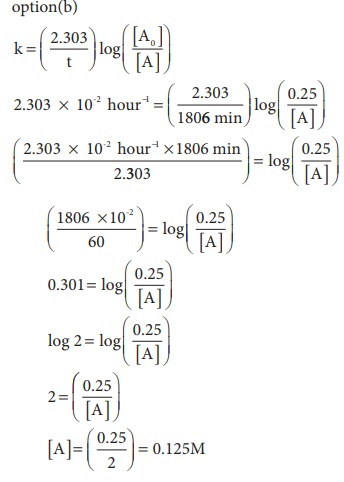13. For a first order reaction, the rate constant is 6.909 min-1.the time taken for 75% conversion in minutes is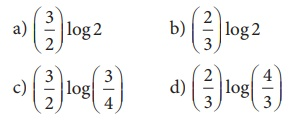Ans: (b)

Solution14. In a first order reaction x →  y ; if k is the rate constant and the initial concentration of the reactant x is 0.1M, then, the half life is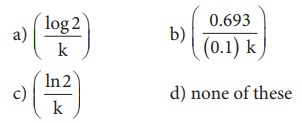Ans: (c)

Solution15. Predict the rate law of the following reaction based on the data given below

2A + B → C + 3Da) rate = k [ A]2 [B]

b) rate = k [ A][B]2

c) rate = k [ A][B]

d) rate = k [ A]1/2 [B]3/2

Solution16. Assertion: rate of reaction doubles when the concentration of the reactant is doubles if it is a first order reaction.

Reason: rate constant also doubles

a) Both assertion and reason are true and reason is the correct explanation of assertion.

b) Both assertion and reason are true but reason is not the correct explanation of assertion.

c) Assertion is true but reason is false.

d) Both assertion and reason are false.

Solution

For a first reaction, If the concentration of reactant is doubled, then the rate of reaction also doubled.

Rate constant is independent of concentration and is a constant at a constant temperature,

17. The rate constant of a reaction is 5.8 × 10−2 s−1 . The order of the reaction is

a) First order

b) zero order

c) Second order

d) Third order

Solution

The unit of rate constant is s-1 and it indicates that the reaction is first order.

18. For the reaction  N2O5(g) → 2NO2 (g) + ½ O2(g), the value of rate of disappearance of N2O5 is given as 6.5 × 10-2 mol L-1 s-1. The rate of formation of NO2 and O2 is given  respectively as

a) ( 3.25 × 102 mol L1s1 ) and (1.3 × 102 mol L1s)

b) (1.3 × 102 mol L1s1 ) and ( 3.25 × 102 mol L1s1 )

c) (1.3 × 101 mol L1s1 ) and ( 3.25 × 102 mol L1s1 )

d) None of these

Solution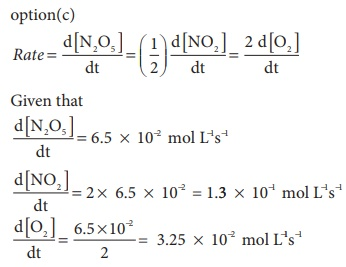19. During the decomposition of H2O2 to give dioxygen, 48 g O2 is formed per minute at certain point of time. The rate of formation of water at this point is

a) 0.75 mol min1

b) 1.5 mol min1

c) 2.25 mol min1

d) 3.0 mol min1

Solutionno of moles of oxygen = (48/32) = 1.5 mol

∴ rate of formation of oxygen = 2 ×1.5 = 3 mol min-1

20. If the initial concentration of the reactant is doubled, the time for half reaction is also doubled. Then the order of the reaction is

a) Zero

b) one

c) Fraction

d) none

Solution

For a first order reaction t1/2 is independent of initial concentration .i.e.,  n ≠ 1; for such cases21. In a homogeneous reaction A →B + C + D , the initial pressure was P0 and after time t it was P. expression for rate constant in terms of P0, P and t will beAns: (a)

Solution22. If 75% of a first order reaction was completed in 60 minutes , 50% of the same reaction under the same conditions would be completed in

a) 20 minutes

b) 30 minutes

c) 35 minutes

d) 75 minutes

Solution

t75% = 2t50%

t50% = (t75% / 2) = (60/2) = 30 min

23. The half life period of a radioactive element is 140 days. After 560 days , 1 g of element will be reduced to

a) (1/2)g

b) (1/4)g

c) (1/8)g

d) (1/16)g

Solution

In 140 days  initial concentration reduced to (1/2)g

In 280 days  initial concentration reduced to (1/4)g

In  420 days  initial concentration reduced to (1/8)g

In 560 days  initial concentration reduced to (1/16)g

24. The correct difference between first and second order reactions is that

a) A first order reaction can be catalysed; a second order reaction cannot be catalysed.

b) The half life of a first order reaction does not depend on [A0]; the half life of a second order reaction does depend on [A0].

c) The rate of a first order reaction does not depend on reactant concentrations; the rate of a second order reaction does depend on reactant concentrations.

d) The rate of a first order reaction does depend on reactant concentrations; the rate of a second order reaction does not depend on reactant concentrations.

Solution

For a first order reaction

t1/2 = 0.6932/k

For a second order reaction25. After 2 hours, a radioactive substance becomes (1/16)th of original amount. Then the half life ( in min) is

a) 60 minutes

b) 120 minutes

c) 30 minutes

d) 15 minutes

Solution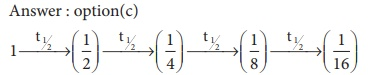4t1/2 = 2 hours

t1/2 = 30 min

Study Material, Lecturing Notes, Assignment, Reference, Wiki description explanation, brief detail
12th Chemistry : UNIT 7 : Chemical Kinetics : Chemical Kinetics: Multiple choice questions with answers |